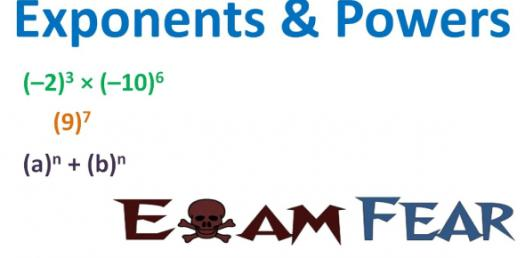# Math Trivia Questions: Powers And Exponent

5 Questions | Attempts: 1545SettingsBelow are a few Math Trivia Questions on Powers And Exponents that is designed to help out students who are having hard time-solving suck problems. The first thing a student should know is how to differentiate the exponential of a given number. Get some practice by taking this quiz and keep an eye out for others that are more practical.

• 1.
A number multiplied by itself.
• A.

Exponenets

• B.

Squared

• C.

Power

• D.

Based

• E.

Cubed

• 2.
The product in which a number used as a factor.
• A.

Cubed

• B.

Based

• C.

Power

• D.

Exponent

• E.

Squared

• 3.
In a power the number of times the base used as a factor
• A.

Power

• B.

Based

• C.

Exponents

• D.

Cubed

• E.

Power

• 4.
A number that can be expressed using an exponenets.
• A.

Squared

• B.

Baesd

• C.

Exponenets

• D.

Power

• E.

Cubed

• 5.
A parallelogram with all sides congruent, all angels, and oppisite sides parallel
• A.

Power

• B.

Based

• C.

Cubed

• D.

Squared

• E.

Square

## Related TopicsBack to top
×

Wait!
Here's an interesting quiz for you.